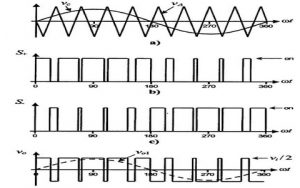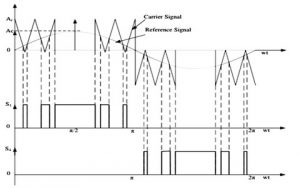Pulse Width Modulated inverters (PWM inverter) replaced the older versions of inverters and has a wide range of applications. Practically these are used in the power electronics circuits. The inverters based on the PWM technology possess MOSFETs in the switching stage of the output. Most of the inverters available nowadays possess this PWM technology and are capable of producing ac voltage for varying magnitudes and frequencies. There are multiple protection and control circuits in these types of inverters. The implementation of PWM technology in the inverters makes it suitable and ideal for the distinct loads connected.

## What is a PWM Inverter?

An inverter whose functionality depends upon the pulse width modulation technology is referred to as PWM inverters. These are capable of maintaining the output voltages as the rated voltages depending on the country irrespective of the type of load connected. This can be achieved by changing the switching frequency width at the oscillator.

### PWM Inverter Circuit Diagram

Circuit diagram of PWM inverter is given in the below diagram

There are various circuits used in the PWM inverters. Some of them are listed below

Battery Charging Current Sensor Circuit
The purpose of this circuit is to sense the current utilized in charging the battery and maintain it at the rated value. It is important to avoid the fluctuations to protect the batteries’ shelf life.

#### Battery Charging Current Sensor Circuit

The purpose of this circuit is to sense the current utilized in charging the battery and maintain it at the rated value. It is important to avoid the fluctuations to protect the batteries’ shelf life.

#### Battery Voltage Sensing Circuit

This circuit is used to sense the voltage required to charge the battery when it is exhausted and begin trickle charging of the battery once it gets fully charged.

#### AC Mains Sensing Circuit

This circuit is to sense the availability of AC mains.  If it is available then the inverter will be in a state of charging and in the absence of mains the inverter will be in battery mode.

#### Soft Start Circuit

It is used to delay the charging for 8 to 10 seconds after resuming the power. It is to protect the MOSFETs from the high currents. This is also referred to as Mains delay.

#### Change Over Circuit

Based on the mains availability this circuit switches the operation of the inverter between the battery and the charging modes.

#### Shut Down Circuit

This circuit is to monitor the inverter closely and shut it down whenever any abnormality incurred.

#### PWM Controller Circuit

To regulate the voltage at the output this controller is used. The circuit needs to perform PWM operations are incorporated in the IC’s and these are present in this circuit.

#### Battery Charging Circuit

The process of charging a battery in the inverter is controlled by this circuit. The output generated by the sensing circuit of the mains and the sensor circuits of the battery is the inputs for this circuit.

#### Oscillator Circuit

This circuit is incorporated with the IC of PWM. It is used to generate the switching frequencies.

#### Driver Circuit

The output of the inverter gets driven by this circuit based on the switching signal of frequency generated.  It is similar to that of a preamplifier circuit.

#### Output Section

This output section comprises a step-up transformer and it is used to drive the load.

### Working Principle

An inverter designing involves various topologies of power circuits and the methods to control the voltage. The most concentrated part of the inverter is its waveform generated at the output. For the purpose of filtering the waveform inductors and the capacitors are used. In order to reduce the harmonics from the output low pass filters are used.

If the inverter possesses a fixed value of output frequencies resonant filters are used. For the adjustable frequencies at the output, filters are tuned above the maximum value of fundamental frequency. PWM technology changes the square wave characteristics. The pulses used for switching are modulated and regulated before it supplied to the connected load. When there is no requirement for voltage control fixed width of the pulse is used.

### PWM Inverter Types & Waveforms

The technique of PWM in an inverter comprises of two signals. One signal is for the reference and the other will be the carrier. The pulse required for switching the mode of the inverter can be generated by the comparison among those two signals. There are various PWM techniques.

#### Single Pulse Width Modulation (SPWM)

For every half cycle, there is only one pulse available to control the technique. The square wave signal will be for reference and a triangular wave will be the carrier. The gate pulse generated will be the result of the comparison of the carrier and the reference signals. Higher harmonics is the major drawback of this technique.

#### Multiple Pulse Width Modulation (MPWM)

MPWM technique is used to overcome the drawback of SPWM. Instead of a single pulse, multiple pulses are used for every half cycle of the voltage at the output. The frequency at the output is controlled by controlling the frequency of the carrier.

#### Sinusoidal Pulse Width Modulation

In this type of PWM technique, instead of a square wave, a sine wave is used as a reference and the carrier will be a triangular wave. The sine wave will be the output and its RMS value of voltage is controlled by the modulation index.#### Modified Sinusoidal Pulse Width Modulation

The carrier wave is applied for the first and the last sixty-degree interval per every half cycle. This modification is introduced to improve the harmonic characteristics. It decreases the loss due to switching and increases the fundamental component.### Applications

Most commonly PWM inverters are utilized in the speed AC drives where the speed of the drive is dependent on the variation in the frequency of the applied voltage. Majorly the circuits in power electronics can be controlled by using PWM signals. To generate the signals in analog form from digital devices like microcontrollers, the PWM technique is beneficial. Further, there are various applications where PWM technology is used in different circuits.

Thus, this is all about an overview of PWM inverter, types, working, and their applications. Can you describe how PWM technology is used in telecommunication?